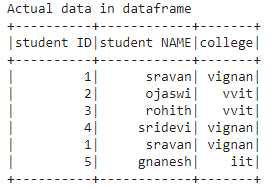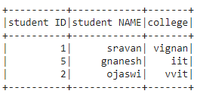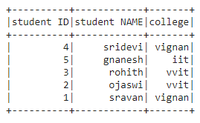# Removing duplicate rows based on specific column in PySpark DataFrame

• Last Updated : 06 Jun, 2021

In this article, we are going to drop the duplicate rows based on a specific column from dataframe using pyspark in Python. Duplicate data means the same data based on some condition (column values). For this, we are using dropDuplicates() method:

Syntax: dataframe.dropDuplicates([‘column 1′,’column 2′,’column n’]).show()

where,

• dataframe is the input dataframe and column name is the specific column
• show() method is used to display the dataframe

Let’s create the dataframe.

## Python3

 `# importing module ` `import` `pyspark ` ` `  `# importing sparksession from pyspark.sql ` `# module ` `from` `pyspark.sql ``import` `SparkSession ` ` `  `# creating sparksession and giving an app name ` `spark ``=` `SparkSession.builder.appName(``'sparkdf'``).getOrCreate() ` ` `  `# list  of students  data ` `data ``=` `[[``"1"``, ``"sravan"``, ``"vignan"``], [``"2"``, ``"ojaswi"``, ``"vvit"``], ` `        ``[``"3"``, ``"rohith"``, ``"vvit"``], [``"4"``, ``"sridevi"``, ``"vignan"``],  ` `        ``[``"1"``, ``"sravan"``, ``"vignan"``], [``"5"``, ``"gnanesh"``, ``"iit"``]] ` ` `  `# specify column names ` `columns ``=` `[``'student ID'``, ``'student NAME'``, ``'college'``] ` ` `  `# creating a dataframe from the lists of data ` `dataframe ``=` `spark.createDataFrame(data, columns) ` ` `  `print``(``'Actual data in dataframe'``) ` `dataframe.show() `

Output:Dropping based on one column

## Python3

 `# remove duplicate rows based on college  ` `# column ` `dataframe.dropDuplicates([``'college'``]).show() `

Output:Dropping based on multiple columns

## Python3

 `# remove duplicate rows based on college  ` `# and ID column ` `dataframe.dropDuplicates([``'college'``, ``'student ID'``]).show() `

Output:My Personal Notes arrow_drop_up
Recommended Articles
Page :